FEATool Multiphysics  v1.15.5 Finite Element Analysis Toolbox
Inductance in Parallel Wires

Calculates the inductance between parallel wires. Two 1 m wires with 4 mm radius 1 A current are placed 0.5 m apart in a large domain of non-conducting air or vacuum. The inductance is computed with both using the magnetic field energy and flux linkage, and compared against the analytical solution L = (µ0/π⋅l⋅(ln(d/r)+1/4)).

This model is available as an automated tutorial by selecting Model Examples and Tutorials... > Electromagnetics > Inductance in Parallel Wires from the File menu. Or alternatively, follow the step-by-step instructions below.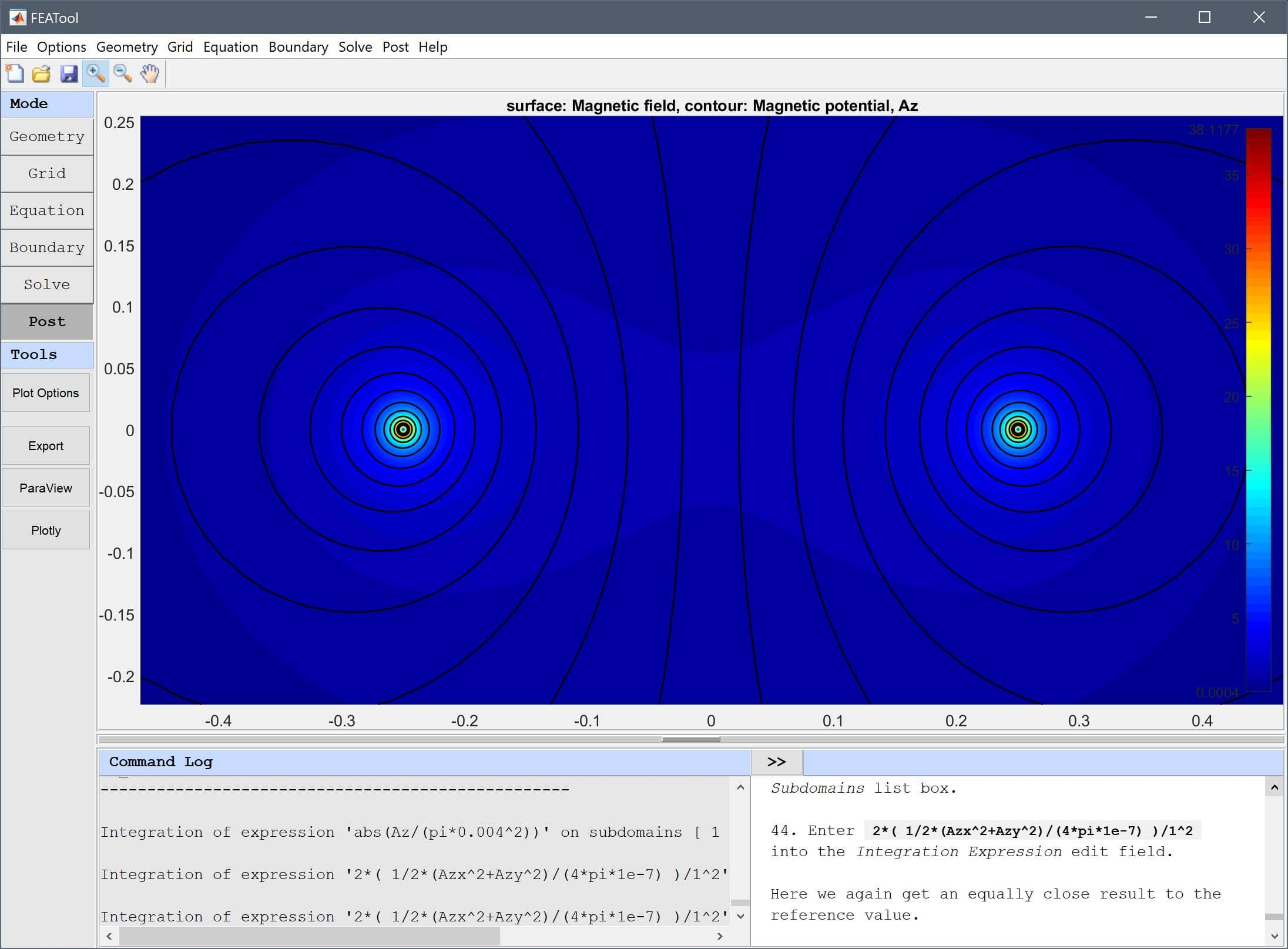# Tutorial

1. To start a new model click the New Model toolbar button, or select New Model... from the File menu.
2. Select the Magnetostatics physics mode from the Select Physics drop-down menu.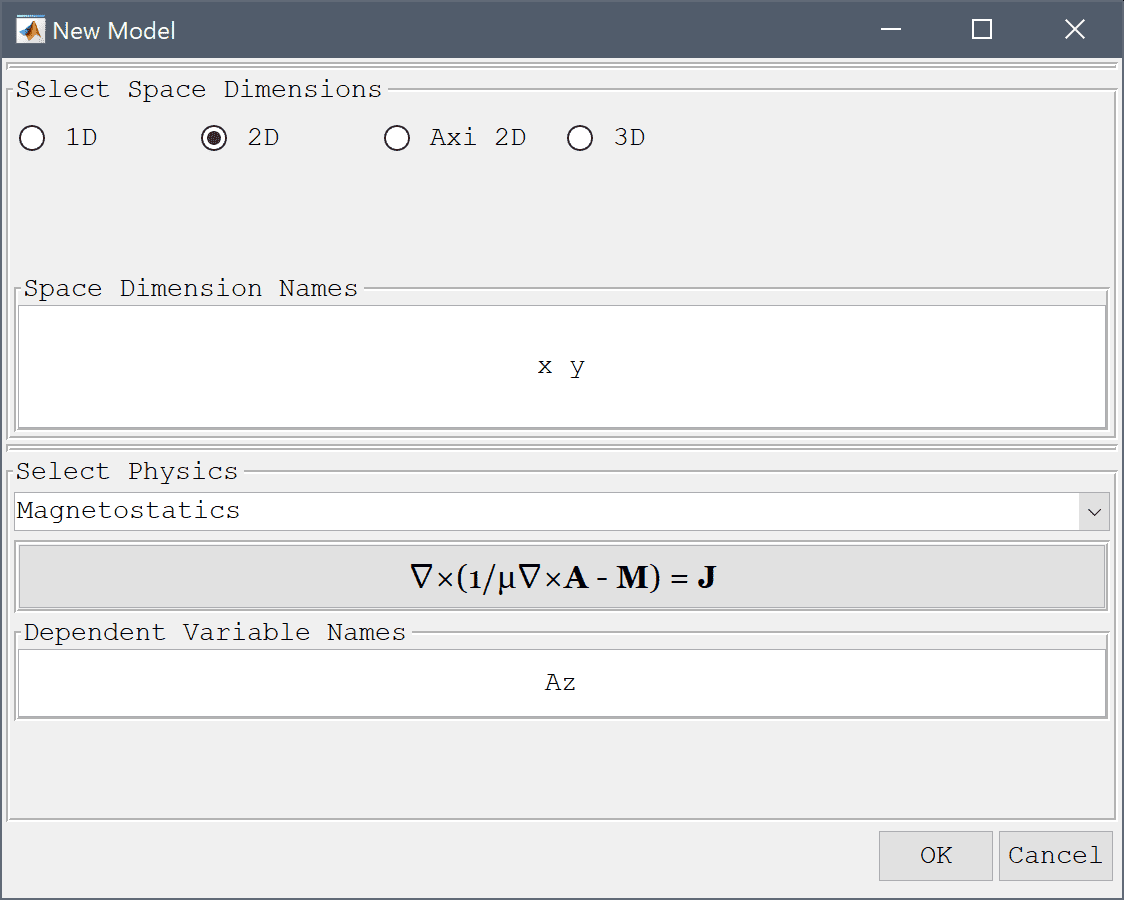3. Press OK to finish the physics mode selection.

The basic domain is a circle centered at (0, 0) with radius 5 m.

1. Select Circle from the Geometry menu.
2. Enter 5 into the radius edit field.
3. Press OK to finish and close the dialog box.

Now create two circles 0.5 m apart each with radius 4 mm for the wire cross sections.

1. Select Circle from the Geometry menu.
2. Enter -0.25 0 into the center edit field.
3. Enter 4e-3 into the radius edit field.
4. Press OK to finish and close the dialog box.
5. Select C2 in the geometry object Selection list box.

The second wire can be created by copying and offsetting the first.

1. Press the Copy and/or transform selected geometry object Toolbar button.
2. Enter 1 into the Number of copies to make edit field.
3. Enter 0.5 0 into the Displacement vector (x and y-components) edit field.
4. Press OK to finish and close the dialog box.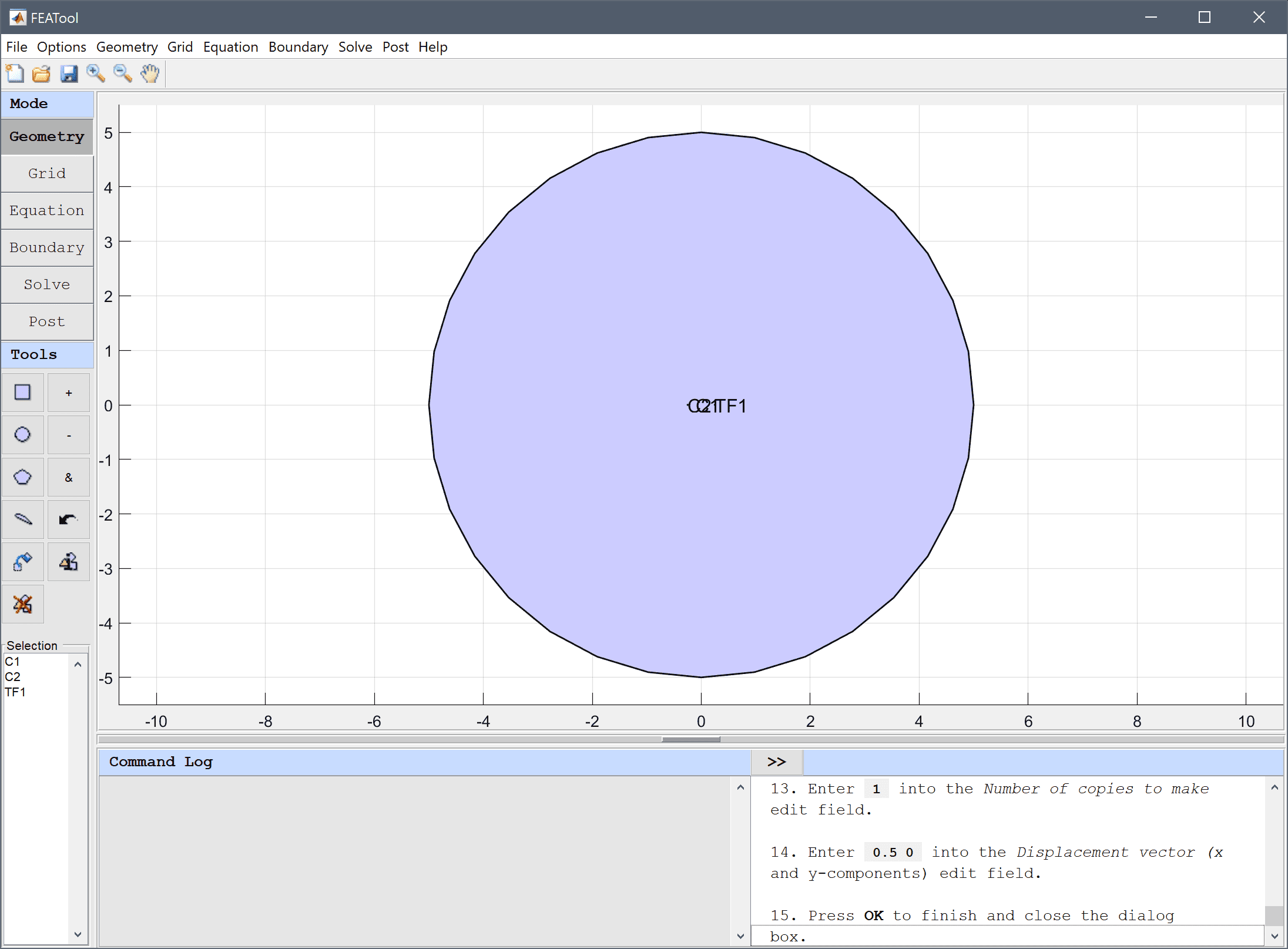5. Switch to Grid mode by clicking on the corresponding Mode Toolbar button.

One can specify mesh sizes per subdomain by entering a space separated list of target mesh sizes in the Grid Size edit field (switch to Subdomain/Equation mode to see the domain order and numbering). Here we use target grid sizes of 0.5e-3 for the two wires and 0.5 for the surrounding medium.

1. Enter 0.5 0.5e-3 0.5e-3 into the Grid Size edit field.
2. Press the Generate button to call the grid generation algorithm.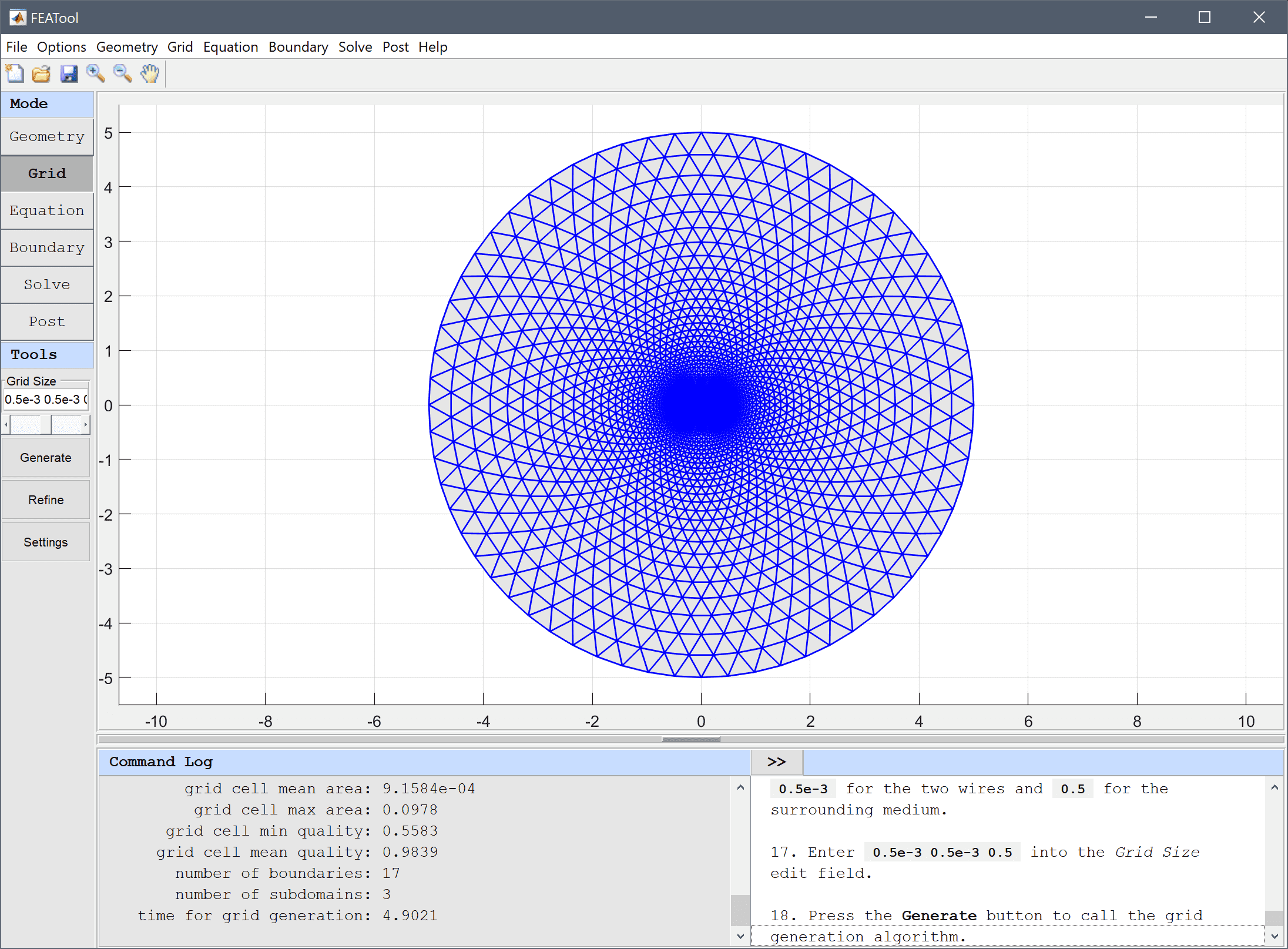3. Press the Equation mode button to switch from grid mode to physics and equation/subdomain specification mode. In the Equation Settings dialog box that automatically opens, set all domains to use a total permeability of 4*pi*1e-7 H/m, and the wires with opposite current densities of 1/(pi*0.004^2) A/m2. Note that FEATool does not enforce any specific unit system, but it is up to the user to select and use consistent units.
4. Select 1 in the Subdomains list box.
5. Enter 4*pi*1e-7 into the Permeability edit field.
6. Enter 0 into the Current density, z edit field.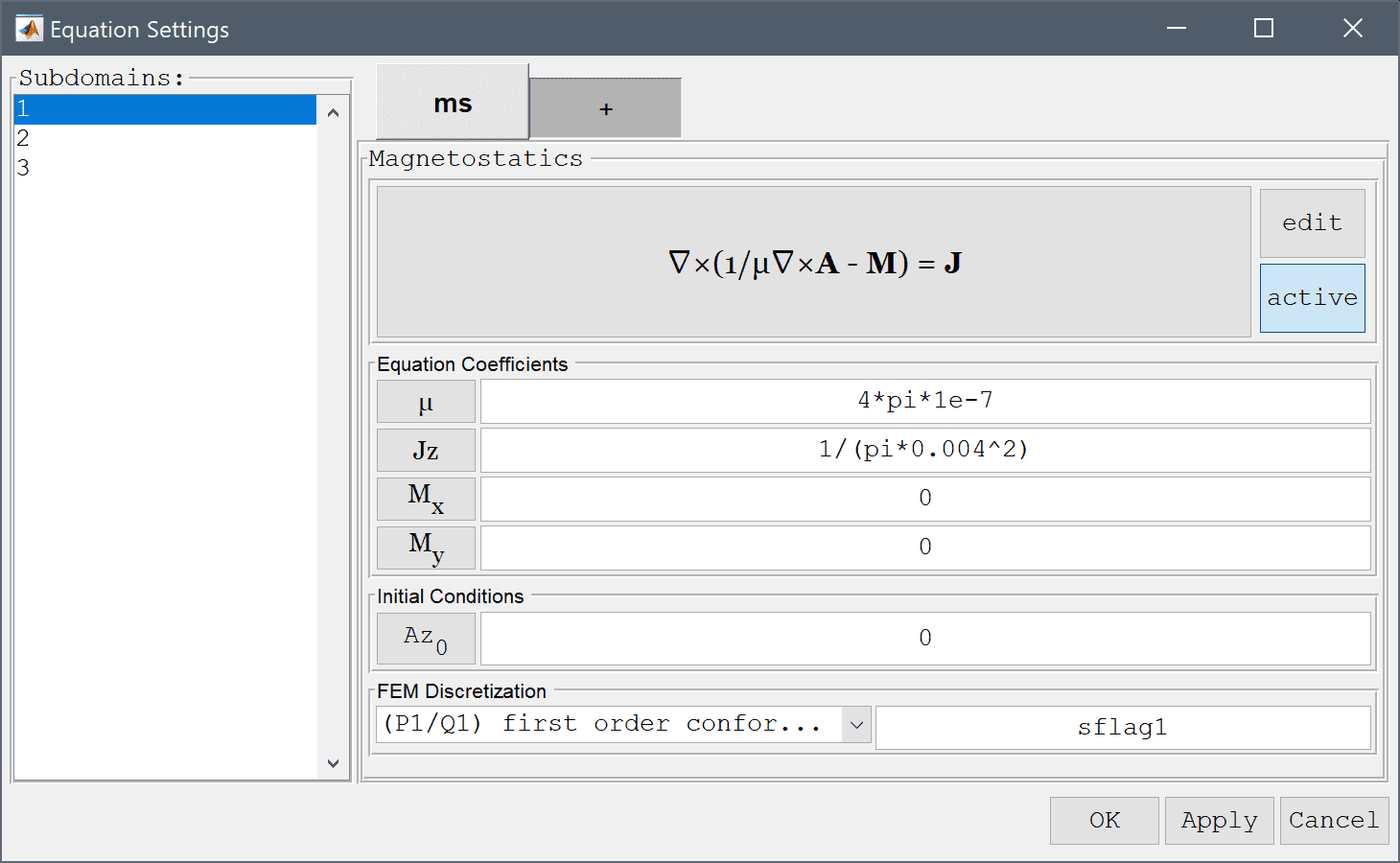7. Select 2 in the Subdomains list box.
8. Enter 4*pi*1e-7 into the Permeability edit field.
9. Enter 1/(pi*0.004^2) into the Current density, z edit field.
10. Select 3 in the Subdomains list box.
11. Enter 4*pi*1e-7 into the Permeability edit field.
12. Enter -1/(pi*0.004^2) into the Current density, z edit field.
13. Press OK to finish the equation and subdomain settings specification.
14. Switch to Boundary mode by clicking on the corresponding Mode Toolbar button.

Select the Magnetic insulation, Az = 0 boundary condition for all the outer boundaries.

1. Select 1, 2, 3, and 4 in the Boundaries list box.
2. Select Magnetic insulation/antisymmetry from the Magnetostatics drop-down menu.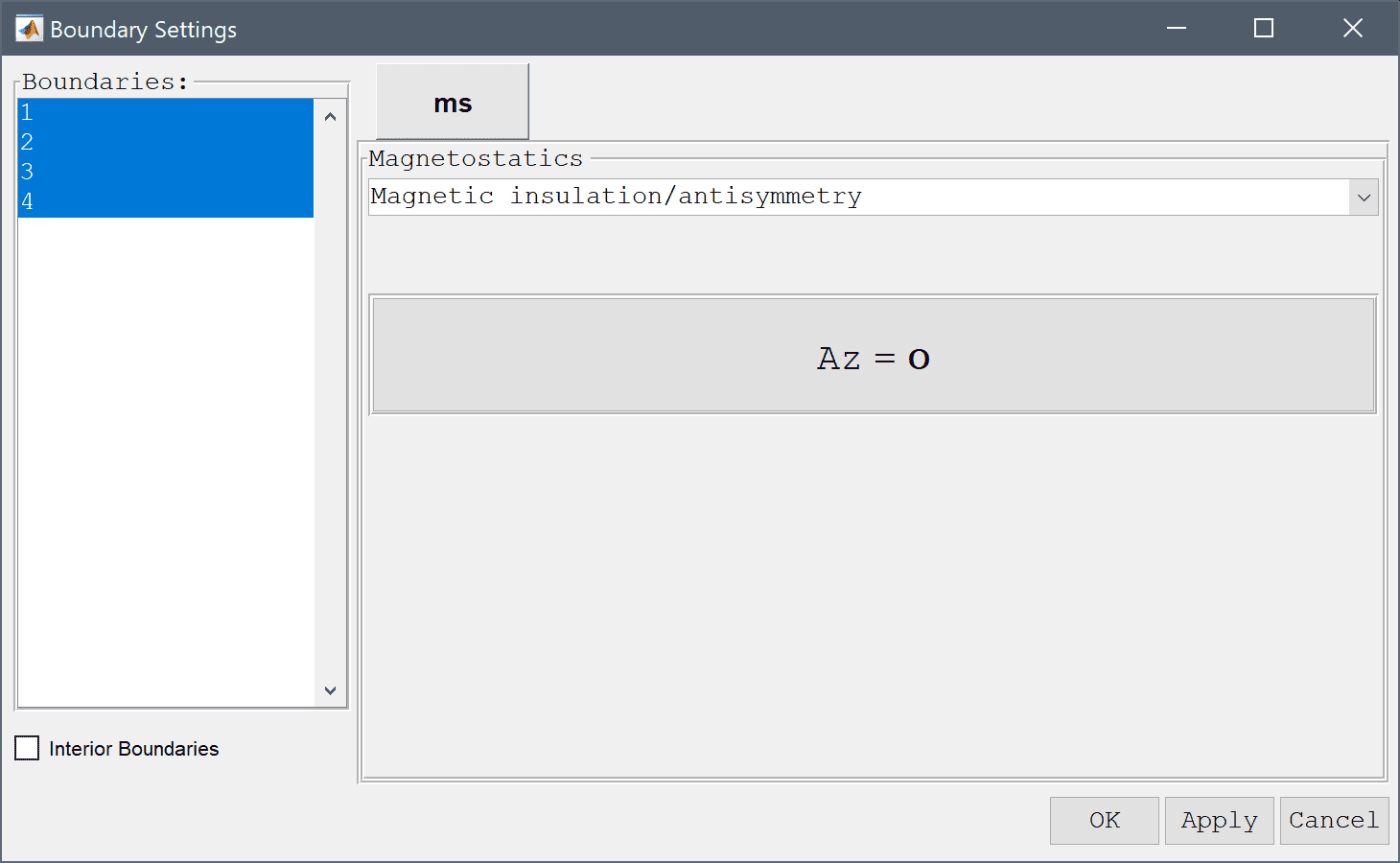3. Press OK to finish the boundary condition specification.
4. Now that the problem is fully specified, press the Solve Mode Toolbar button to switch to solve mode. Then press the = Tool button to call the solver with the default solver settings.

Select and plot the Magnetic potential, Az with contour lines and use the zoom button in the upper toolbar to zoom in on the wires.

1. Press the Plot Options Toolbar button.
2. Select the Contour Plot check box.
3. Enter 30 into the Number or specified vector of contour levels to plot edit field.
4. Press OK to plot and visualize the selected postprocessing options.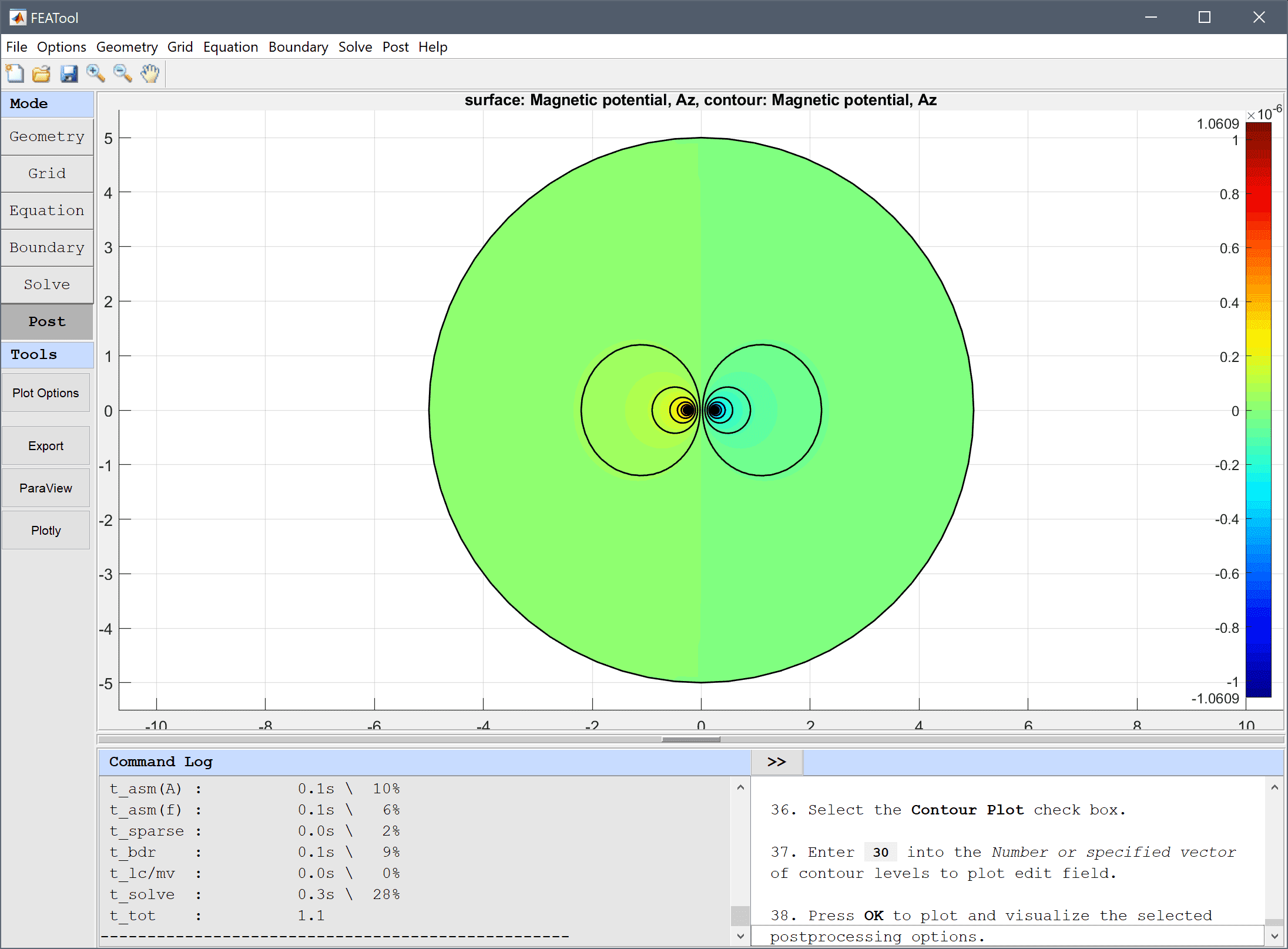To compare the accuracy of the solution we first calculate the flux linkage by integrating the magnetic potential abs(Az/(pi*0.004^2)) per area over the wires, and dividing by the current 1 A.

1. Select Subdomain Integration... from the Post menu.
2. Select 1 and 2 in the Subdomains list box.
3. Enter abs(Az/(pi*0.004^2)) into the Integration Expression edit field.
4. Press the Apply button.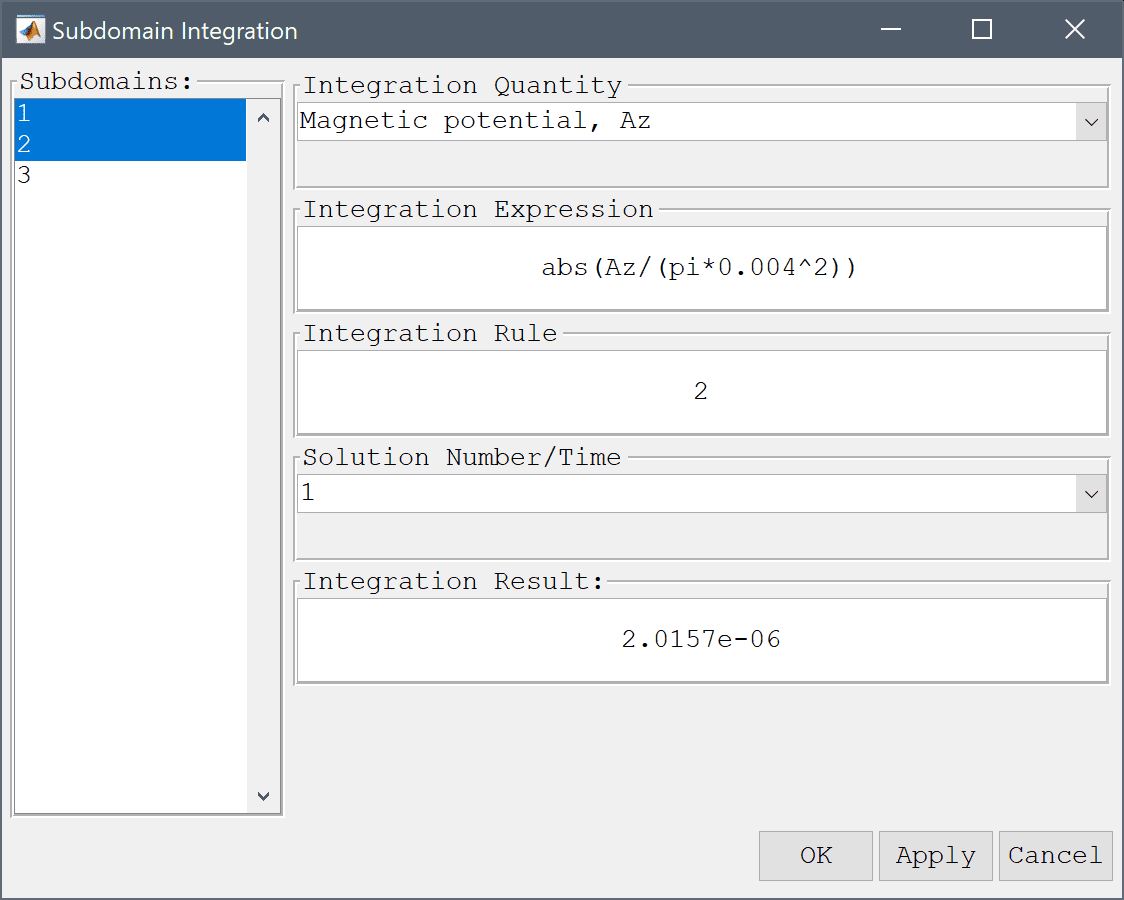This results in a inductance value of 2.0157e-6 H which compares very well with the theoretical value of 2.0313e-06 H.

Another way to compute the inductance is to first calculate the total magnetic field energy W = 1/2∫(BE)dV so that the inductance is given by L = 2W/I2. This is done in the following steps.

1. Select 1, 2, and 3 in the Subdomains list box.
2. Enter 2*( 1/2*(Azx^2+Azy^2)/(4*pi*1e-7) )/1^2 into the Integration Expression edit field.

Here we again get an equally close result to the reference value.

1. Press the Apply button.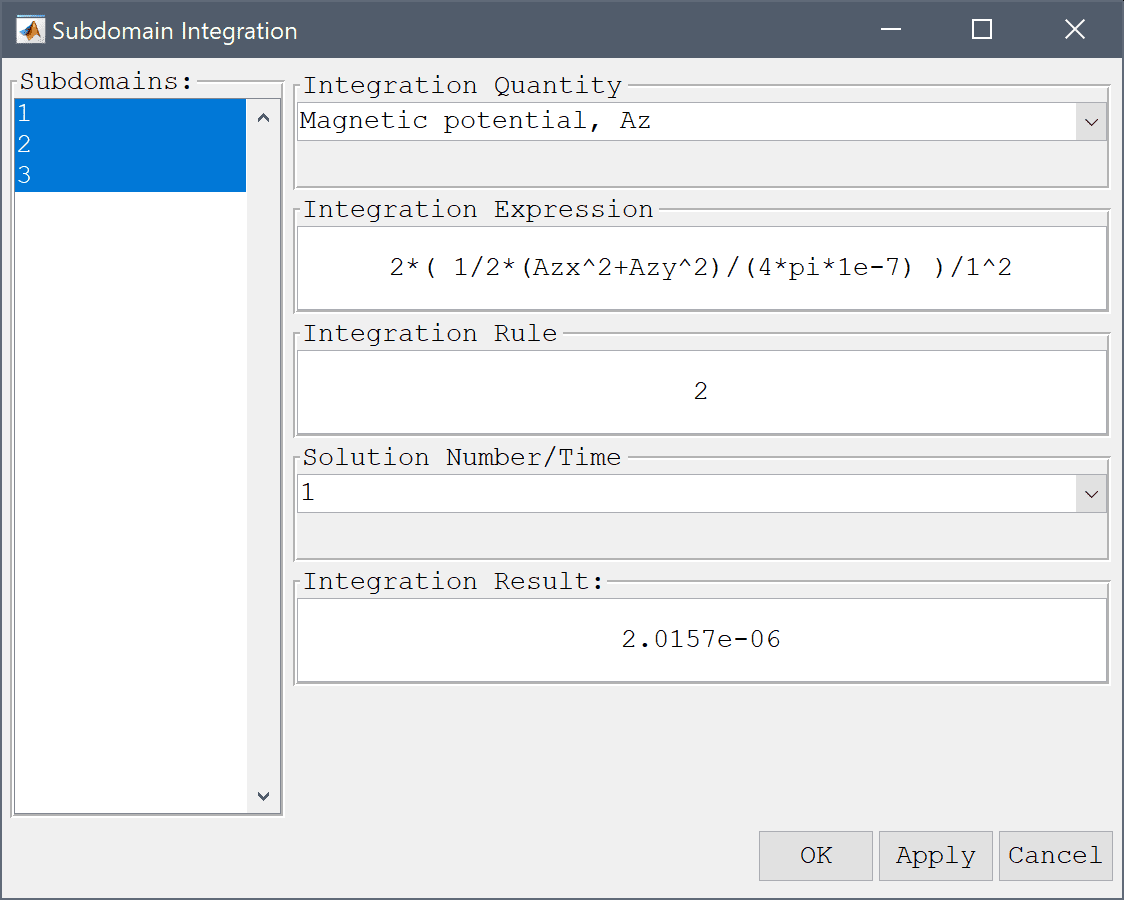2. Press OK to finish and close the dialog box.

The inductance in parallel wires electromagnetics model has now been completed and can be saved as a binary (.fea) model file, or exported as a programmable MATLAB m-script text file, or GUI script (.fes) file.